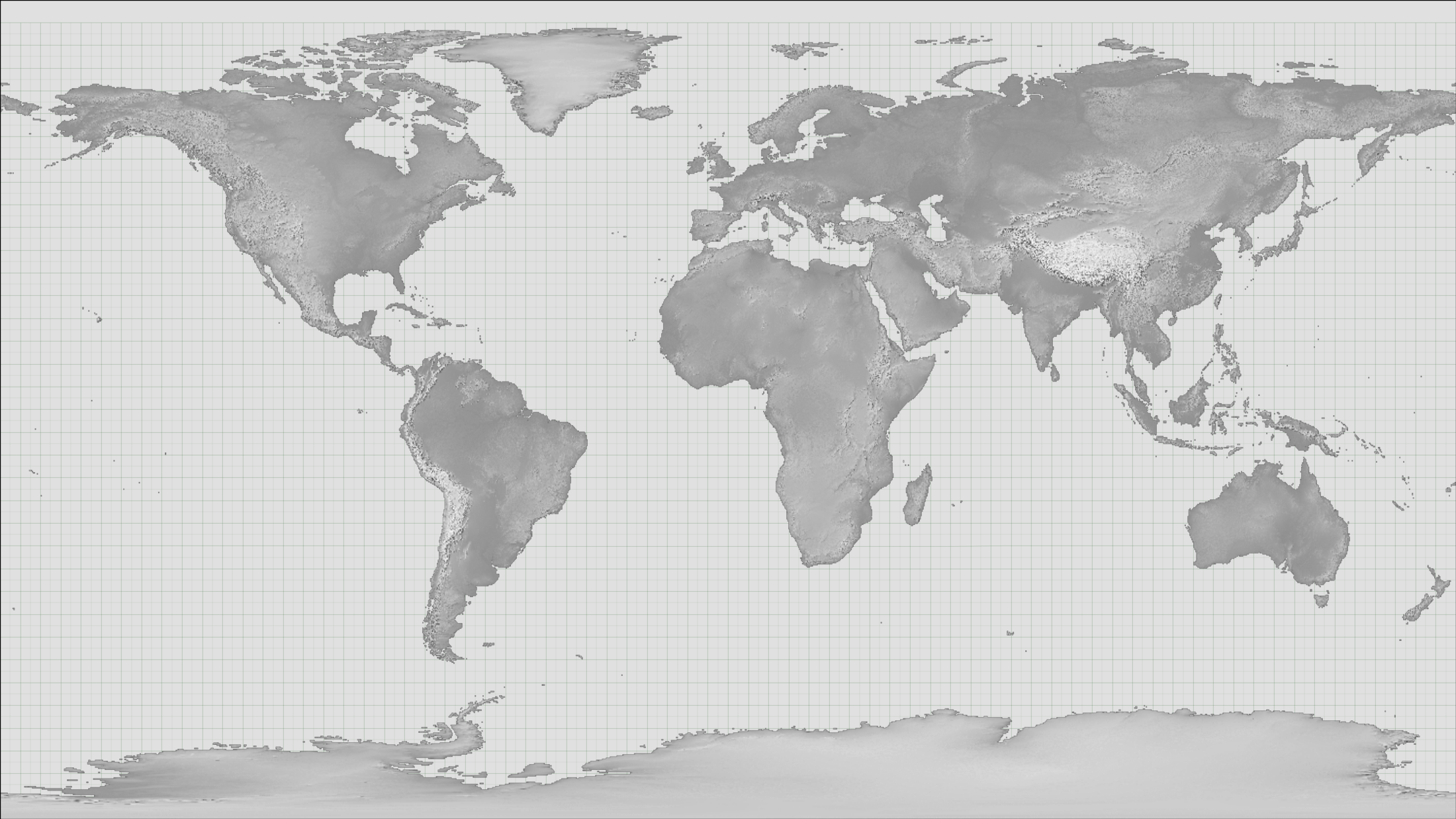# Meteorographica examples: map background¶Background (continents and lat:lon grid) for weather plots.

```# Meteorographica example script

# Set up the figure and add the continents as background
# (don't actually plot any weather).

import Meteorographica as mg

import matplotlib
from matplotlib.backends.backend_agg import FigureCanvasAgg as FigureCanvas
from matplotlib.figure import Figure
import cartopy
import cartopy.crs as ccrs

# Define the figure (page size, background color, resolution, ...
aspect=16/9.0
fig=Figure(figsize=(22,22/aspect),              # Width, Height (inches)
dpi=100,
facecolor=(0.88,0.88,0.88,1),
edgecolor=None,
linewidth=0.0,
frameon=False,                # Don't draw a frame
subplotpars=None,
tight_layout=None)
# Attach a canvas
canvas=FigureCanvas(fig)

# All mg plots use Rotated Pole, in this case just use the standard
#  pole location.
projection=ccrs.RotatedPole(pole_longitude=180.0, pole_latitude=90.0)

# Define an axes to contain the plot. In this case our axes covers
#  the whole figure
ax.set_axis_off() # Don't want surrounding x and y axis
# Set the axes background colour
ax.background_patch.set_facecolor((0.88,0.88,0.88,1))

# Lat and lon range (in rotated-pole coordinates) for plot
extent=[-180.0,180.0,-90.0,90.0]
ax.set_extent(extent, crs=projection)
# Lat:Lon aspect does not match the plot aspect, ignore this and
#  fill the figure with the plot.
matplotlib.rc('image',aspect='auto')

# Draw a lat:lon grid